# Excel: Discover How To Use Functions

You may have heard a lot about functions in Microsoft Excel, but you have no idea what they look like or how to use them. Functions are awesome, that's what makes Excel so powerful. In this short tutorial you will learn everything you need to know about functions.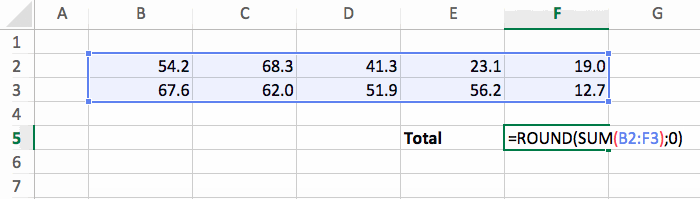## The Theory

In an Excel file, you can add a function in any cell by typing something like this:

`=FUNC_NAME(param1; param2)`

Let's analyze everything here.

• Equal sign: a function is a formula, so you have to start it with an `=` at the beginning.
• Name: the name of the function in uppercase that have already been defined in Excel.
• Brackets: after the name we have to use `()` to put the parameters inside.
• Parameters: there can be any number of parameters, and they are separated by semicolon or comas (depending on the setup of your Excel). There are 5 main types of parameters: number (42), text ("hello"), boolean (TRUE or FALSE), cell address (D4), and range address of cells (D4:E12).

Note that when typing a function you don't have to put the name in uppercase. This means that you can type `=func_name(param1; param2)` and Excel will automatically convert that into `=FUNC_NAME(param1; param2)`.

Sounds a bit confusing? Let's see some examples of Excel functions to make things more concrete!

## The ROUND Function

Let's start with a really simple function: ROUND. As its name implies, it will round a number. And it looks like this:

`=ROUND(cell; number_of_digits)`

It has 2 parameters:

• cell: the cell we want to round.
• number_of_digits: how many digits do we want to display after the decimal point.

So if we have the number 3.1415 in the cell B3, and we want to display 3.14 (that's 2 digits after the the decimal point), we should write `=ROUND(B3; 2)`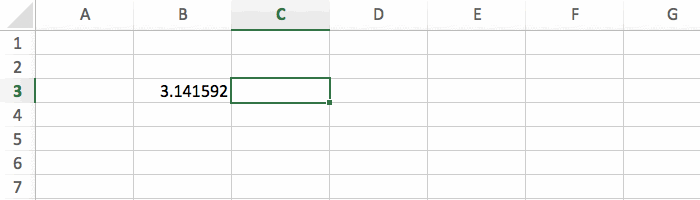And here's how it looks like with different values for the number_of_digits parameter.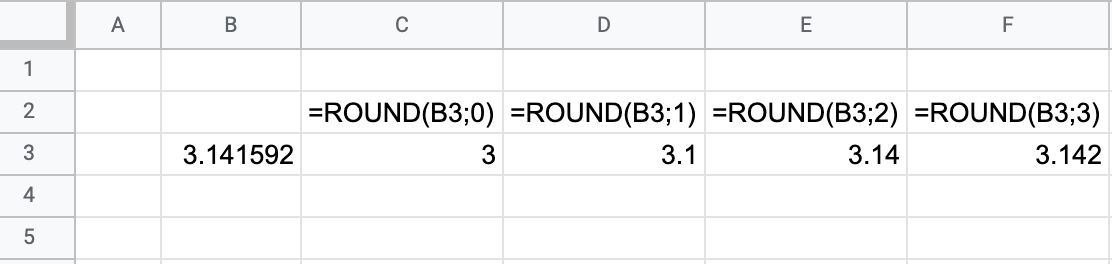There are sometimes some restrictions to respect when using the cell or range as a parameter in a function. For example, the cell used in the first parameter of the ROUND function has to be a number value. If ROUND is applied to a cell of text, the error #VALUE! will occur. You can learn more about this in the tutorial six common error messages you can get in Excel.

## The Function

The SUM function is probably the most frequently used functions in Excel. We use it to make the sum of a range of cells. Here's the formula:

`=SUM(range)`

This function has only one parameter: the range of cells that we want to sum.

You can see how to use it in the example below with `=SUM(B2:F3)`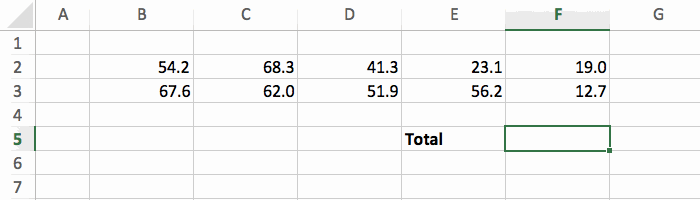You can either select the cells with the arrow keys (like I did), with the mouse, or by directly typing B2:F3. It's up to you.

## Nested Functions

Functions become even more interesting when you combine them.

Using the examples above, we could do this `=ROUND(SUM(B2:F3); 0)` to have the sum rounded.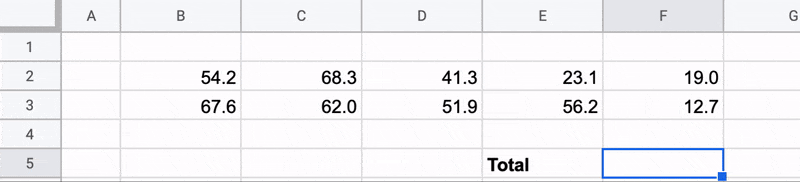Note that the order in the nested functions is important. Doing `=SUM(ROUND(B2:F3; 0))` wouldn't work because ROUND doesn't work with a range as a parameter.

## Conclusion

Once you understand how these basics functions work, you can start using more complex one (like COUNT, IF, VLOOKUP, etc.) or even create your own functions. That will unlock you the true power of Excel :-)

Here's a quick summary of what we covered:

• General syntax: `=FUNC_NAME(param1; param2)`
• ROUND function: `=ROUND(cell; number_of_digits)`
• SUM function: `=SUM(range)`